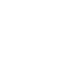# SAT Question of the Day (ACT too!): Apr. 28, 2014

If you are reading this in an email you received from me, do not click the link to sat.collegeboard.org below. Use the link to my website that is farther down on the email.

http://sat.collegeboard.org/practice/sat-question-of-the-day?questionId=20140428&oq=1 (This link takes you to today’s question. If you use my archive, you will see the question related to my SAT explanation for that date.)

The answer is C.  This is a simple definition question.  Do you know what “undefined” means?  If so, this question is simple.  If not, then you will have a hard time with it.  “Undefined” simply means you are trying to divide by zero.  That means if there is a 0 in the denominator the fraction is “undefined.”  However, you can always have a 0 in the numerator.  For example, 7/0 is undefined but 0/7 mean you have “zero sevenths,” which means you have zero.

For this question, because x can be any number, it could be equal to c.  That means the denominator would be 0 when x = c because x c = 0 when x and c are equal.

When you have zero in the numerator, f can still be defined.  That means Roman Numerals I and II are wrong answers.

I have included many of the common math terms in my materials and you should review them in the Number Properties I and II lessons.  However, it is impossible to list all the possible terms.  Be sure you practice using actual SAT and ACT questions and if there are terms that you don’t understand, please send me an email and I will define them for you.  More importantly, I will add that term to my list because I’m sure other students will have trouble as well.

Let’s see what the ACT folks have for us today.

The answer is J.  This is a great question to use to review ratio questions even though it is about a triangle.  Whenever you see a ratio question, add up the parts.  In this case 2x + 3x + 5x = 10x.  The sum (10x in this case) represents the whole.  The smallest angle is 2x/10x or 1/5 of the whole.  The three angles in a triangle add up to 180 degrees.  Therefore, 180/5 = 36, Answer J.

Something to remember is that the sum of the parts doesn’t always represent the whole.  However, the whole or the entire population will always be a multiple of the sum of the parts.  For example, a triangle that has a ratio of angles that are 1x:2x:3x, with a sum of 6x, certainly has more than 6 degrees!  Divide 6 into 180 degrees (the whole or population) and you get x = 30.  So, this is a 30:60:90 right triangle.

This is a significant special triangle because it appears on the test quite frequently.  You need to read what I have to say about it on my free website or watch the Special Triangles lesson in Video Series 700.  There are special ratios of the lengths of the side that are on the SAT “cheat sheet.”  It is half of an equilateral triangle.  Watch for x√3 for one of the sides (not hypotenuse) of a right triangle.  It will almost certainly mean you have a 30:60:90 triangle and you can use the ratio of the sides 1:2:√3 to determine the lengths of the sides and hypotenuse.  You won’t need to use the Pythagorean Theorem.   These will be a huge clue regarding a time-saving shortcut.

QotD Words of “Wiz-dom”:

Let’s continue with series on the Pillars of Test-Taking “Wiz-dom.”

Pillar V: The Answer Is On the Page:

This is my favorite.  Never forget that on multiple-choice questions, you don’t necessarily have to create the right answer; you just have to find it!  It will be right in the test booklet and you just need to eliminate the other answers.  That makes the test-writers’ puzzles almost fun!

For the reading test, you are forced to find the right/best answer by eliminating others that can look awfully good!  You can use my PICK strategy to make this process much easier.  The answers to reading passage questions have certain, specific, predictable characteristics.  Right answers have certain traits and wrong answers have certain traits.  Learn them by reading my free website or viewing the Attack the Questions lesson on Video Series #300.

For the math test, whenever you see a question that says something like, “what is the value of x,” or “solve for y,” one of the answers has to be x or yStart with Answer C and plug-in the answers.  If it is too big, try the smaller answers and, conversely, if it is too small, try bigger answers.  By starting with C (which will be the middle-sized answer because they will be in ascending or descending order), you can eliminate three answers by checking out only one answer!  That will certainly save you lots of time.

You have 5 days before the test.  Be sure you schedule some time to practice.

If you are in my Haiku class, be sure to start reviewing your Worksheets.  They are an invaluable tool.

Bob Alexander, the “SAT and ACT Wizard”